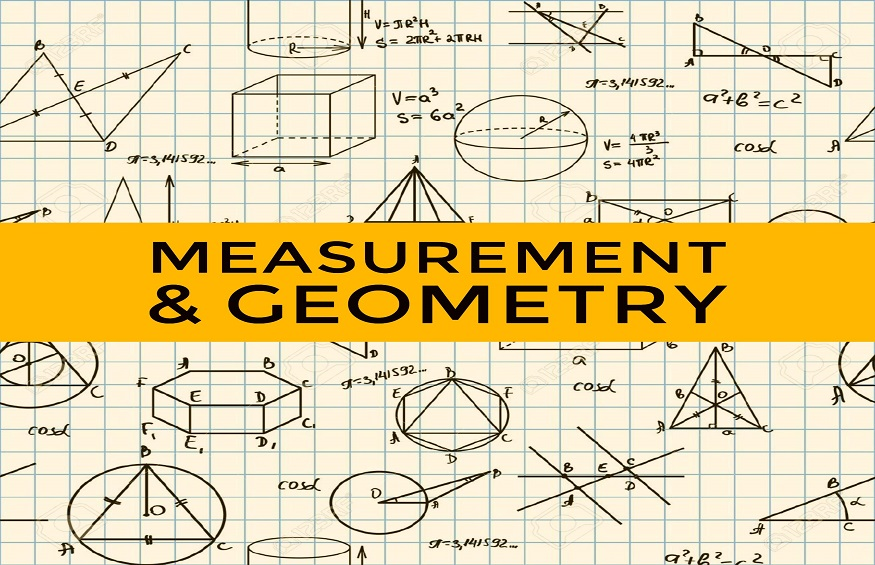1,757 Views

Students can learn many mathematical skills during their schooling. Finding the measurements of geometric shapes is one of the most important skills that students should become perfect at. To master these skills, one should follow some basic guidelines and equations to remember the formulas forever. To accomplish this task, students will require right information and should be able to perform problem solving techniques efficiently. We know that geometry is involved in our daily existence everywhere hence, we need to know some basic measurements of geometric shapes.

Geometric shapes can be categorized into two types; two-dimensional shapes and three-dimensional shapes. Two-dimensional shapes have length, width, perimeter and area as their measurements, whereas three dimensional shapes have volume including the surface areas, perimeter of bases. For example, circumference of circle, area of a rectangle are related to 2D figures and volume of a sphere, surface area of a cube are related to 3D figures.

Now, the question is how to measure these parameters for different geometric shapes. In case of 2D figures, perimeter can be found by measuring the distance around the shape, area can be found by measuring the area enclosed by the given shape. All these measurements will help us in performing real life calculations. For example, in order to design the door of a house, first we have to determine the length and width of the door that has to fit exactly. In this case, we can use the rectangular shape formulas.

Generally speaking, measurements involve assigning numbers to the characteristics of shapes or figures such that those numbers result in reality in the form of perimeter, area, volume and so on. Also, these measurements differ from one shape to another, based on the type of geometric shape. But, there is one special case with respect to the triangular shape. We know that there are different types of triangles which are defined based on the sides and angles. For these triangles, the perimeter formula is still the same, i.e. adding all the three sides.